# CBSE Class 12 Vectors Algebra

• ### NCERT Solutions Class 12 Maths Vector Algebra

Maths Vector Algebra  Exercise 10.1 Question 1: Represent graphically a displacement of 40 km, 30° east of north. Answer Here, vector OP represents the displacement of 40 km, 30° East of North.   Question 2: Classify the following measures as scalars and vectors. (i) 10 kg (ii) 2 metres north-...

• ### CBSE Class 12 Mathematics Vector Algebra Assignment Set D

Please click the below link to access CBSE Class 12 Mathematics Vector Algebra Assignment Set D

• ### CBSE Class 12 Mathematics Vector Algebra Worksheet

CBSE Class 12 Mathematics Worksheet - Vector Algebra. CBSE issues sample papers every year for students for class 12 board exams. Students should solve the CBSE issued sample papers to understand the pattern of the question paper which will come in class 12 board exams this year. The sample papers...

# CBSE Class 12 Mathematics HOTs Vectors Set C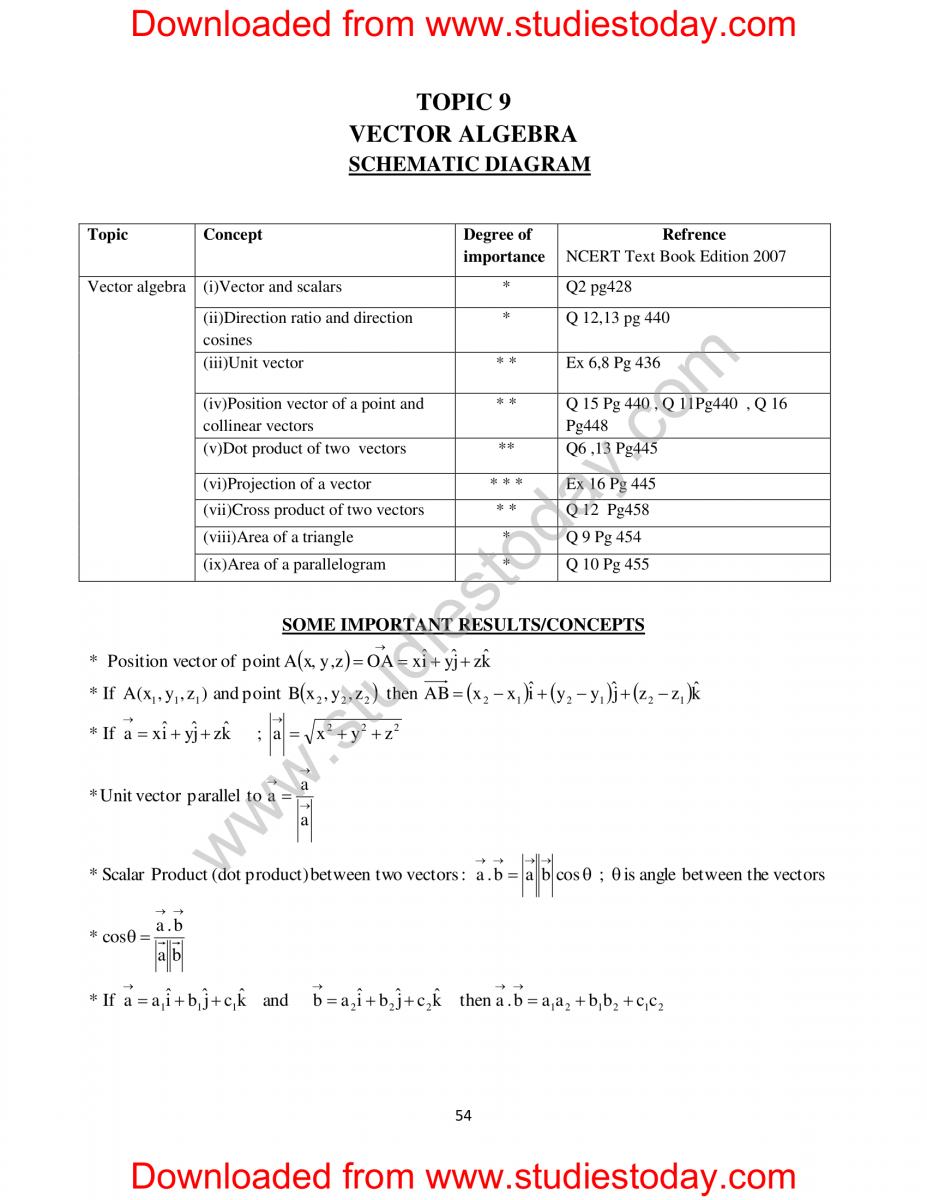Click for more Vectors Algebra Study Material
 CBSE Class 12 Mathematics HOTs Vectors Set C CBSE Class 12 Mathematics HOTs Vectors Set B CBSE Class 12 Mathematics HOTs Vectors Set A

# NCERT Solutions Class 12 Maths Vector Algebra

Maths Vector Algebra

Exercise 10.1

Question 1: Represent graphically a displacement of 40 km, 30° east of north.Click for more Vectors Algebra Study Material
 NCERT Solutions Class 12 Maths Vector Algebra

# CBSE Class 12 Mathematics Vectors And 3D Notes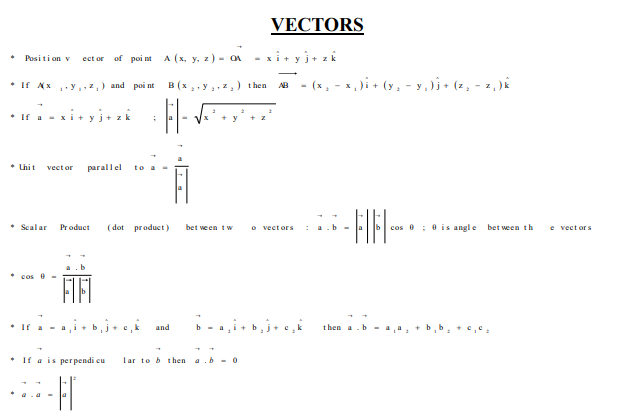Click for more Vectors Algebra Study Material
 CBSE Class 12 Mathematics Vectors And 3D Notes

# CBSE Class 12 Mathematics Vector Algebra Assignment Set D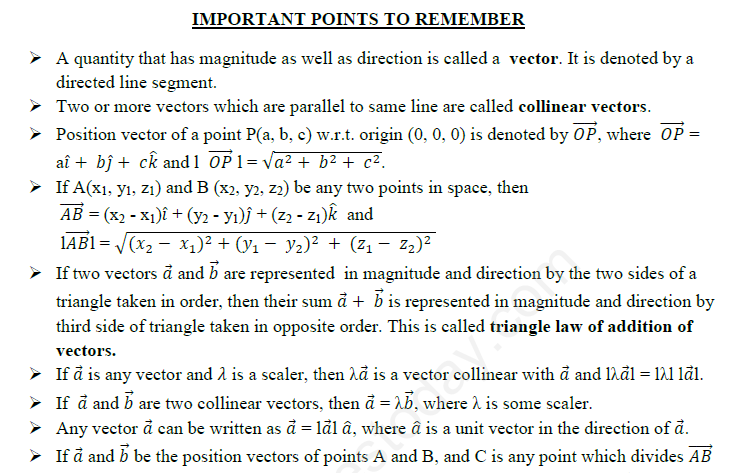Click for more Vectors Algebra Study Material
 CBSE Class 12 Mathematics Vector Algebra Assignment Set C CBSE Class 12 Mathematics Vector Algebra Assignment Set D CBSE Class 12 Mathematics Vector Algebra Assignment Set A CBSE Class 12 Mathematics Vector Algebra Assignment Set B

# CBSE Class 12 Mathematics HOTs Vectors Set A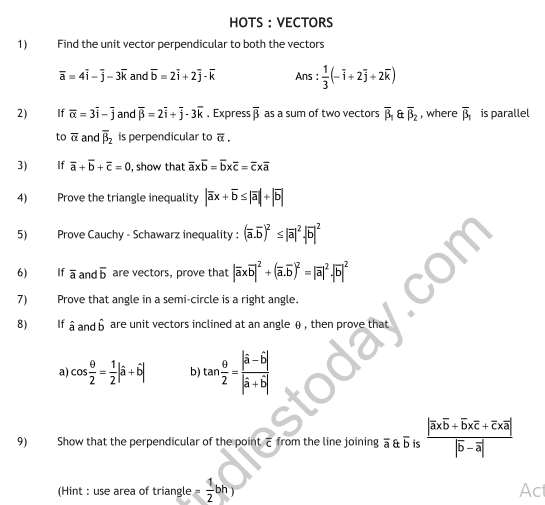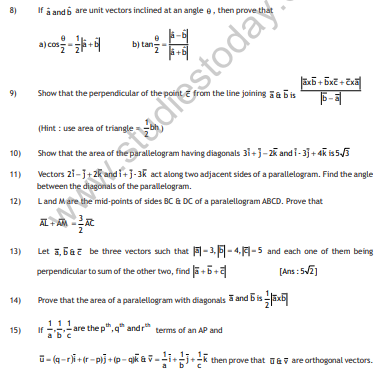Click for more Vectors Algebra Study Material
 CBSE Class 12 Mathematics HOTs Vectors Set C CBSE Class 12 Mathematics HOTs Vectors Set B CBSE Class 12 Mathematics HOTs Vectors Set A

# CBSE Class 12 Mathematics Vector Algebra Assignment Set CClick for more Vectors Algebra Study Material
 CBSE Class 12 Mathematics Vector Algebra Assignment Set C CBSE Class 12 Mathematics Vector Algebra Assignment Set D CBSE Class 12 Mathematics Vector Algebra Assignment Set A CBSE Class 12 Mathematics Vector Algebra Assignment Set B

## Latest NCERT & CBSE News

Read the latest news and announcements from NCERT and CBSE below. Important updates relating to your studies which will help you to keep yourself updated with latest happenings in school level education. Keep yourself updated with all latest news and also read articles from teachers which will help you to improve your studies, increase motivation level and promote faster learning